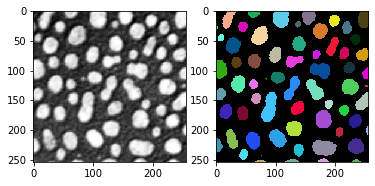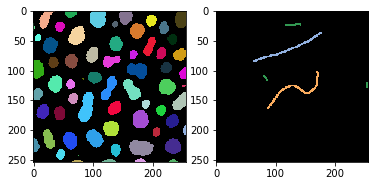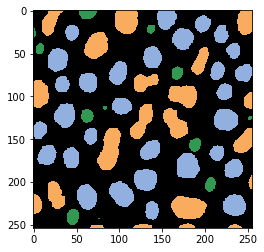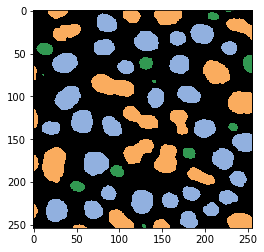# Object classification on OpenCL-compatible GPUs#

APOC is based on pyclesperanto and scikit-learn. It allows classifying objects according to measured properties / features such as intensity, shape and number of neighboring cells.

import apoc

import pyclesperanto_prototype as cle
import numpy as np
import matplotlib.pyplot as plt


For object classification, we need an intensity image and a label image as input.

# load intensity image

# segment the image
labels = cle.label(cle.threshold_otsu(image))

fig, axs = plt.subplots(1, 2)

cle.imshow(image, color_map="Greys_r", plot=axs)
cle.imshow(labels, labels=True, plot=axs)## Training#

We also need a ground truth annotation image. This image is also a label image with a sparse annotation. A line with value 1 was drawn through all objects that are supposed to belong to class 1. A line with value 2 was drawn through all objects that should be classified as class 2. If the line crosses the background, this is ignored. In this example, objects were annotated in three classes:

• Elongated objects

• Roundish objects

• Small objects

annotation = cle.push(imread('../../data/label_annotation.tif'))

fig, axs = plt.subplots(1, 2)

cle.imshow(labels, labels=True, plot=axs)
cle.imshow(annotation, labels=True, plot=axs)Next, we need to define which features we want to use for classifying objects. We will use area, shape and the standard deviation of the intensity.

features = 'area mean_max_distance_to_centroid_ratio standard_deviation_intensity'

# Create an object classifier
filename = "../../data/blobs_object_classifier.cl"
classifier = apoc.ObjectClassifier(filename)

# train it; after training, it will be saved to the file specified above
classifier.train(features, labels, annotation, image)


After the classifier has been trained, we can use it immediately to predict the classification of the objects in the image.

# determine object classification
classification_result = classifier.predict(labels, image)

cle.imshow(classification_result, labels=True)## Prediction#

You can also reload the classifier from disc and apply it to other images. We will simulate this by rotating the original image. This is by the way a good sanity check to see if the classification depends on the orientation of the image.

image2 = cle.rotate(image, angle_around_z_in_degrees=90)
labels2 = cle.rotate(labels, angle_around_z_in_degrees=90)

classifier2 = apoc.ObjectClassifier("../../data/blobs_object_classifier.cl")

classification_result2 = classifier2.predict(labels2, image2)

cle.imshow(classification_result2, labels=True)## Available features for object classification#

We can print out all available features . Parameters with a ? expect a number at that position and can be specified multiple times with multiple values.

apoc.list_available_object_classification_features()

['label',
'original_label',
'bbox_min_x',
'bbox_min_y',
'bbox_min_z',
'bbox_max_x',
'bbox_max_y',
'bbox_max_z',
'bbox_width',
'bbox_height',
'bbox_depth',
'min_intensity',
'max_intensity',
'sum_intensity',
'area',
'mean_intensity',
'sum_intensity_times_x',
'mass_center_x',
'sum_intensity_times_y',
'mass_center_y',
'sum_intensity_times_z',
'mass_center_z',
'sum_x',
'centroid_x',
'sum_y',
'centroid_y',
'sum_z',
'centroid_z',
'sum_distance_to_centroid',
'mean_distance_to_centroid',
'sum_distance_to_mass_center',
'mean_distance_to_mass_center',
'standard_deviation_intensity',
'max_distance_to_centroid',
'max_distance_to_mass_center',
'mean_max_distance_to_centroid_ratio',
'mean_max_distance_to_mass_center_ratio',
'touching_neighbor_count',
'average_distance_of_touching_neighbors',
'average_distance_of_n_nearest_neighbors=?',
'average_distance_of_n_nearest_neighbors=?']